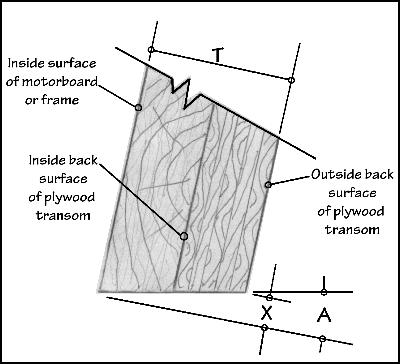# Formula for figuring finished transom sizeThe transom pattern perimeter is generally given to the outside back surface, see sketch above. However many boats have transoms angled (A) aft, in the case of outboards about 12° to 15°. Thus the angle required on the bottom, and usually the sides, requires more material (X) than the pattern outline. Cutting the angle away from or greater than the given pattern contour solves the problem. Or, the extra material required for the angle at any given point can be obtained. The sketch shows the total thickness (T) of the transom plus the motor board or frame. The sketch above can be duplicated using the angle given on the plans and the total thickness of the transom. Distance "X", the amount of additional material required larger than the pattern, can be measured. Optionally X can be obtained by simple arithmetic.

AS A FORMULA: X = Angle factor (A, as listed in chart) multiplied by T (thickness)
X = A x T

As an example solve X for a 1 1/2" thick transom and motorboard or frame with a 12° angle.
X = .213 x 1.5 = .3195"

To find the answer in sixteenths of an inch divide by .0625.
X in 1/16ths =.3195 ÷ .0625 = 5.04 or 5/16"

CHART
Angle in degrees"A"Angle Factor
10 .176
12 .213
13 .231
14 .249
15 .268
16 .287